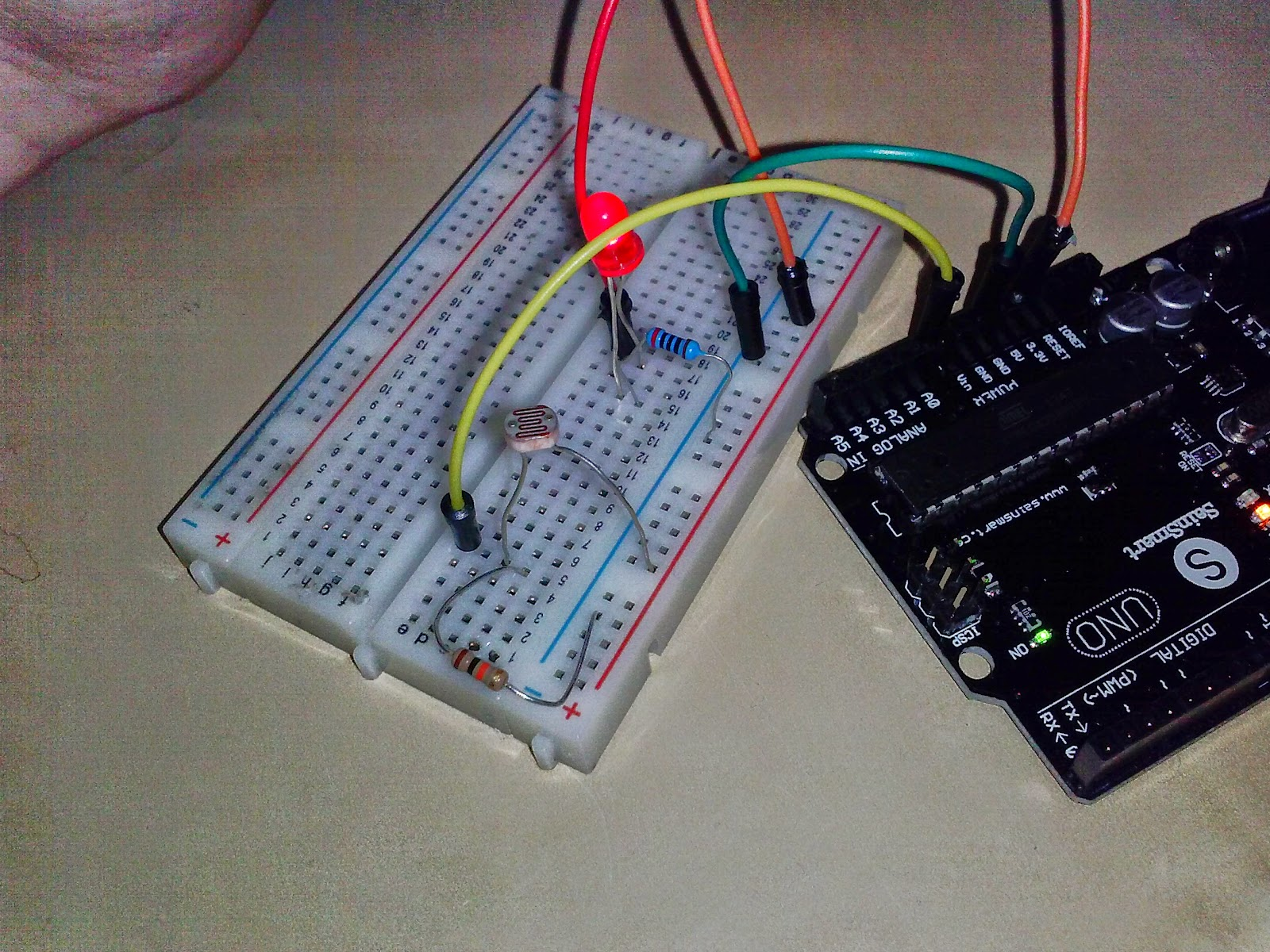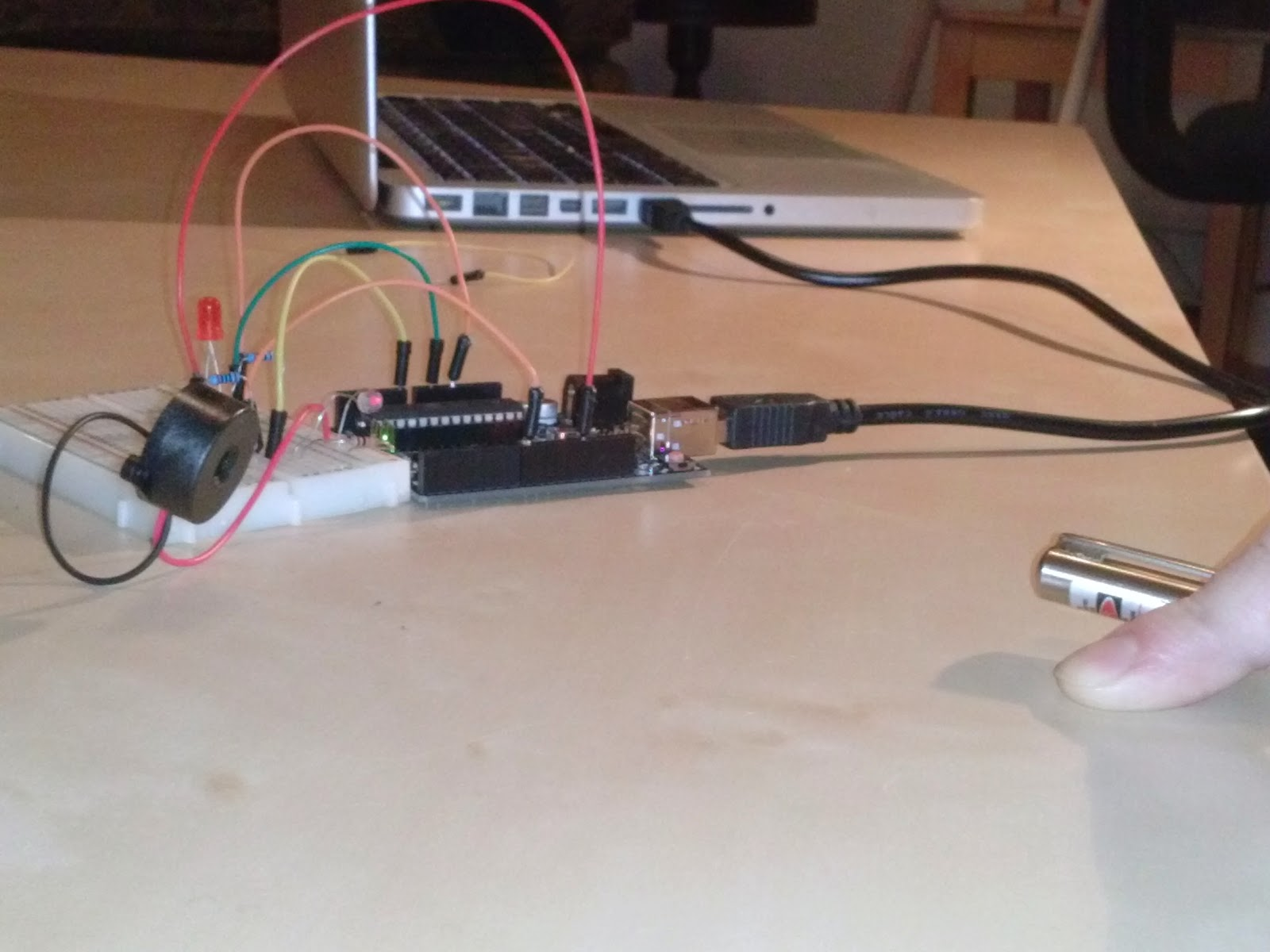bonkerfield

## RASER Week 9: Laser activated trip wire

After last week's success with the physical trip wires, it's time to move on to some serious spy trip wire technology: LASERS!This project combines some of the tricks we've already learned as well as introducing a new concept called analog input. Check out the finished product in action in this video. Warning: the buzzer is a little loud and annoying so maybe turn your sound down before you play it.

#### Connecting the light sensor

The first step is to hook up the light sensor so we can see how analog voltage works. The circuit takes electricity from the 5V pin and runs it through the light sensor into pin A0. There's also another resistor between pin A0 and GND. This basic circuit is called a voltage divider, and the schematic looks like this.We''l learn more about how the voltage divider is working later, but for now just try to hook it up like in this picture.Upload the ReadAnalogVoltage code to the Arduino and open the Serial Monitor to see what voltage is being read on pin A0.

Now, wave your hand above the sensor. Do you notice the voltage value changing on the Serial Monitor? The analog input pins let you measure how much light is shining on the sensor.

In the past weeks, we've focused solely on digital inputs. Digital inputs tell you whether there is at least a certain amount voltage on the pin. The cutoff is normally somewhere around 2 volts. If you hook up any voltage higher than that, the Arduino will view the pin as ON (or HIGH).

Analog works differently. With analog input, the value of the voltage is read into the Arduino. So if you hook up 2 volts, the Arduino will read 2 volts. And if you hook up 1.67 volts, the Arduino will read 1.67.

This is really useful when you have a sensor like the one we're using. The analog input lets us measure continuous changes in the reading on the sensor. So when the light level changes a little bit, the voltage also changes by a little bit. Therefore, we can use the Arduino to measure subtler changes than we could with digital input.

##### The Voltage Divider Circuit

Here's a little conundrum: The Arduino pins measure voltages, but our sensor doesn't actually produce any voltage itself. Instead the sensor changes its resistance when light shines on it.

To convert this change in resistance into a change in voltage, we use a simple circuit called a voltage divider. This circuit is specially designed to cause a change in resistance to trigger a change in the voltage at the midpoint of the circuit. We'll use this circuit repeatedly whenever we need a sensor so it's important to remember how it works.

Now we have the sensor controlling the voltage on pin A0. Next, we want to use the voltage input to control whether an LED will go off. Start by hooking up an LED to pin 13 just like we did for the old physical trip wire.We'll use almost the same code that we used in the physical trip wire from last week. We'll need to modify it so that instead of asking whether there is any voltage on the digital pin, we're going to ask HOW MUCH voltage is coming in on the analog pin..

`int led1 = 13;int led2 = 12;// the setup routine runs once when you press reset:void setup() {  // initialize serial communication at 9600 bits per second:  Serial.begin(9600);  pinMode(led1, OUTPUT);  pinMode(led2, OUTPUT);}// the loop routine runs over and over again forever:void loop() {  // read the input pin:  int sensorValue = analogRead(A0);  float voltage = sensorValue * (5.0 / 1023.0);  Serial.println(voltage);  if(voltage < _______){    digitalWrite(led1,HIGH);    digitalWrite(led2,HIGH);    delay(500);    digitalWrite(led1,LOW);    digitalWrite(led2,LOW);    delay(500);    digitalWrite(led1,HIGH);    digitalWrite(led2,HIGH);    delay(500);    digitalWrite(led1,LOW);    digitalWrite(led2,LOW);    delay(500);    digitalWrite(led1,HIGH);    digitalWrite(led2,HIGH);    delay(500);    digitalWrite(led1,LOW);    digitalWrite(led2,LOW);    delay(500);    digitalWrite(led1,HIGH);    digitalWrite(led2,HIGH);    delay(500);    digitalWrite(led1,LOW);    digitalWrite(led2,LOW);    delay(500);  }  delay(1);        // delay in between reads for stability}`

Above is the code we'll need to make it work. BUT, I've left one spot blank. That's the place where we have to decide what the threshold voltage should be. To figure out what the value should be, point the laser at the light sensor and look at the numbers being printed in the Serial Monitor. You want the threshold to be somewhere between the voltage when the laser is shining and the voltage when the laser is off.

After you upload the code, the LED should blink until you point a laser pointer at it. Then if at any point someone steps in the way, the voltage will change so that the blinking LED loop will get triggered. And that's a trip wire!#### Bonus round! Adding the buzzer

What we have now is called a silent alarm. It activates a light but doesn't sound an alarm to catch your attention.

To bring the alarm to the next level, I also added a buzzer that goes off when the LED goes off. You can use this code and hook up a speaker to make it work. I've just added a single line that controls a tone emitted on pin 8.

`    tone(8,600,3000);`

See if you can figure out where to put this line of code to make the buzzer go off when the LED blinksIn the end, the completed laser trip wire will look like this. Now have some fun catching intruders!

### Discussion Around the Web

Join the Conversation